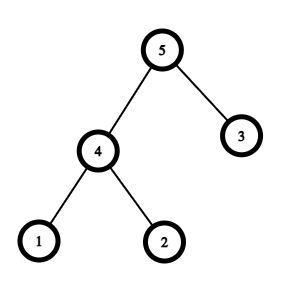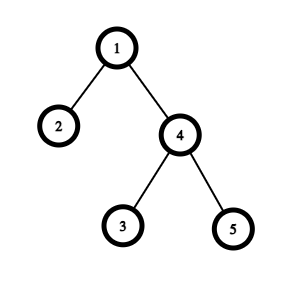# Upside Down Binary Tree

Posted: 26 Mar, 2021
Difficulty: Moderate

## PROBLEM STATEMENT

#### You are given the 'ROOT' of the binary tree. Your task is to return the new 'ROOT' after turning the tree upside down.

##### For Example:
``````Tree = [5, 4, 3, 1, 2, -1, -1, -1, -1, -1, -1]
```````````` Upside down Tree :
````````````ANS = [1, 2, 4, -1, -1, 3, 5, -1, -1, -1, -1]
``````
##### Input Format:
``````The first line contains an Integer 'T' which denotes the number of test cases or queries to be run. Then the test cases follow.

The first line of each test case contains the elements of the tree in the level order form separated by a single space.

If any node does not have a left or right child, take -1 in its place. Refer to the samples below.
``````
##### Output Format:
``````For each test case, output the upside-down binary tree.
Output for each test case will be printed in a separate line.
``````
##### Note:
``````You don’t need to print anything. It has already been taken care of. Just implement the given function.
``````
##### Constraints:
``````1 <= T <= 100
1 <= N <= 5000
1 <= node.values <= 10 ^ 9

Time Limit: 1 second
``````Approach 1

We will first recursively solve the left subtree and then, recursion will return the rightmost node of the upside-down tree of the left subtree, then we will add the right child of the current node to the left of the returned rightmost node. And the current node to the right of the returned node.

After this current node becomes the rightmost node of the upside-down tree and we will return it. The new root node will be the left-most node in the given tree and we will return it in the recursion.

The algorithm will be:

1. recursiveTraversal (node):
1. If (node.left == NULL)
1. return node, node
2. rightMostChild, ans = recursiveTraversal(node.left)
3. rightMostChild.left = node.right
4. rightMostChild.right = node
5. node.left = None
6. Node.right = None
7. return node, ans
2. upsideDownTree(root) :
1. node , ans =  recursiveTraversal(root)
2. return ans